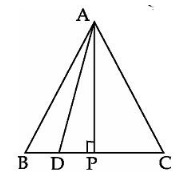QuestionAnswers

# In an equilateral triangle ABC, D is a point on the side BC such that $BD = \dfrac{1}{3}BC$, then show that $9A{D^2} = 7A{B^2}$.Verified
130.2k+ views
Hint: First, we will form the triangle according to given conditions, then draw an altitude in it to use pythagorean theorem such that it contains the sides BD and BC so that we can use the given condition $BD = \dfrac{1}{3}BC$ and thus find the result.

Let us draw the figure first as shown below:Here, ABC is the given triangle and $BD = \dfrac{1}{3}BC$. Let P be a point on BC such that AP is the altitude and we have drawn AD as well because we need the condition $9A{D^2} = 7A{B^2}$ which contains AD.
Consider the $\vartriangle APC$ and $\vartriangle APB$:
$AB = AC$ (Since the triangle is equilateral)
$AP = AP$ (Common)
$\angle B = \angle C$ (Both are ${60^ \circ }$ because of being angles in equilateral triangle)
Hence, $\vartriangle APC \cong \vartriangle APB$ (By SAS property)
SAS property states that two triangles are congruent if they have a pair of equal corresponding angles and two pairs of corresponding equal sides.
Hence, we have $BP = PC$ (By C.P.C.T) …..(1)
$BP + PC = BC = AB$
Using (1), we have:
$BP = PC = \dfrac{1}{2}BC = \dfrac{1}{2}AB$ ……(2)
CPCT stands for Corresponding parts of Congruent triangles. CPCT theorem states that if two or more triangles which are congruent to each other are taken then the corresponding angles and the sides of the triangles are also congruent to each other.
Let us now first get to know the pythagorean theorem:
It says that if we have a right angled triangle, right angled at B, then $A{C^2} = A{B^2} + B{C^2}$
Now consider the $\vartriangle ABP$:-
Applying pythagorean theorem on it, we get:
$A{B^2} = A{P^2} + P{B^2}$
This implies that $A{P^2} = A{B^2} - P{B^2}$
Using (2), we will get:-
$\therefore A{P^2} = A{B^2} - {\left( {\dfrac{1}{2}AB} \right)^2}$
$\therefore A{P^2} = A{B^2} - \dfrac{1}{4}A{B^2}$
$\therefore A{P^2} = \dfrac{{4A{B^2} - A{B^2}}}{4} = \dfrac{{3A{B^2}}}{4}$ …….(3)
Now, consider $\vartriangle ADP$:-
Applying pythagorean theorem on it, we get:
$A{D^2} = A{P^2} + P{D^2}$ ……(4)
Applying (3) in (4), we get:-
$A{D^2} = \dfrac{3}{4}A{B^2} + D{P^2}$ …..(5)
We clearly see that $BD + DP = BP$
So, we have: $DP = BP - BD$
Putting this in (5), we will get:-
$A{D^2} = \dfrac{3}{4}A{B^2} + {(BP - BD)^2}$ …….(6)
We are given that $BD = \dfrac{1}{3}BC$ and since ABC is an equilateral triangle which means $AB = BC$.
This implies $BD = \dfrac{1}{3}BC = \dfrac{1}{3}AB$ …….(7)
Using (1) and (7) in (6), we get:
$A{D^2} = \dfrac{3}{4}A{B^2} + {(\dfrac{1}{2}AB - \dfrac{1}{3}AB)^2}$
Simplifying RHS:
$A{D^2} = \dfrac{3}{4}A{B^2} + \dfrac{1}{{36}}A{B^2}$
Simplifying the RHS further:
$A{D^2} = \dfrac{3}{4}A{B^2} + \dfrac{1}{{36}}A{B^2} = \dfrac{{27 + 1}}{{36}}A{B^2} = \dfrac{{28}}{{36}}A{B^2}$
Cross multiplying:
$36A{D^2} = 28A{B^2}$
Cutting 4 from both sides:
$9A{D^2} = 7A{B^2}$
Hence, we have proved the required expression.

Note: The students may make the mistake of applying the pythagorean theorem on the triangles ABD and ACD but, you must always remember that, we need a right angled triangle to apply the pythagorean theorem.